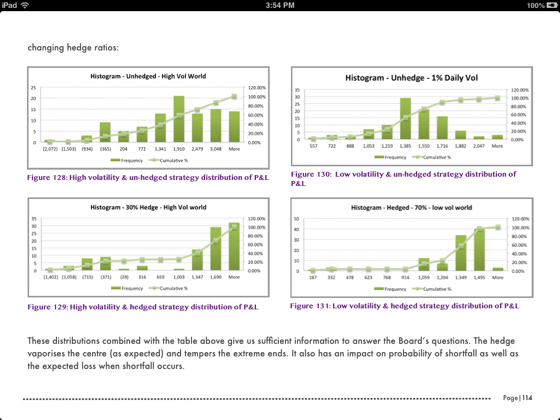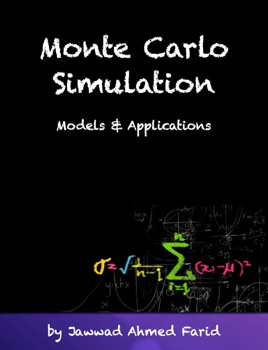• \$27.99

## Publisher Description

This guide to Monte Carlo Simulation covers:

Building Monte Carlo simulation models in EXCEL for equities, commodities and currencies

Building a hybrid Monte Carlo simulation model that uses the actual historical return distribution instead of the normal distribution assumption used in the original version

Option pricing using a Monte Carlo Simulation Model

Using the MC model to explain Black Scholes risk adjusted probabilities
Pricing a European Call option using an MC simulator

Convergence and Variance Reduction Techniques for option pricing models

Antithetic Variable Technique
Quasi Random Sequences

Further applications of Monte Carlo Simulation models

Pricing exotic options
Calculating the Value at Risk measure for futures and options
Monte Carlo simulation VaR using Historical Simulation
Monte Carlo simulation How-to reference – Fuel Hedging problem
Jet Fuel Aviation Hedging Case Study
Simulating the term structure of interest rates
Forecasting the monetary policy rate

Also included are:

8 instructor led videos that walk you through steps for building a Monte Carlo simulation model, and using the model to understanding the Black Scholes equation as well as price vanilla and exotic options

5 review session to test the reader’s understanding of the material

•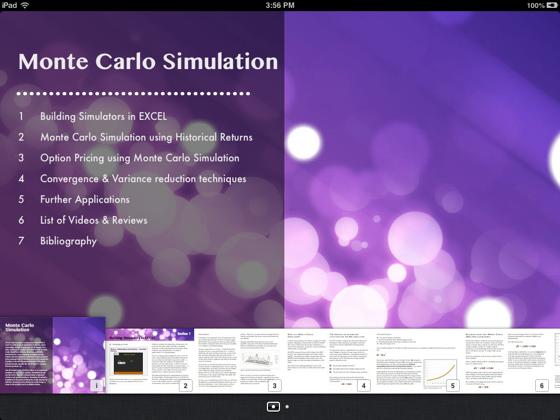•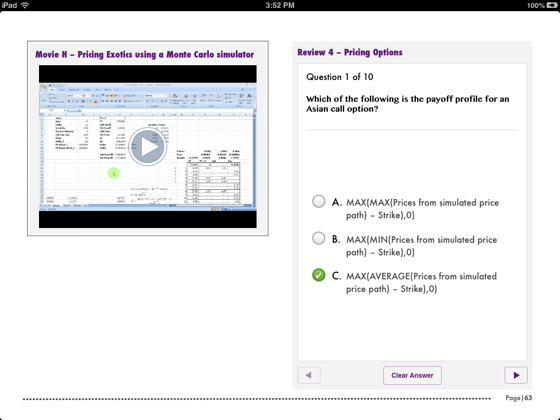•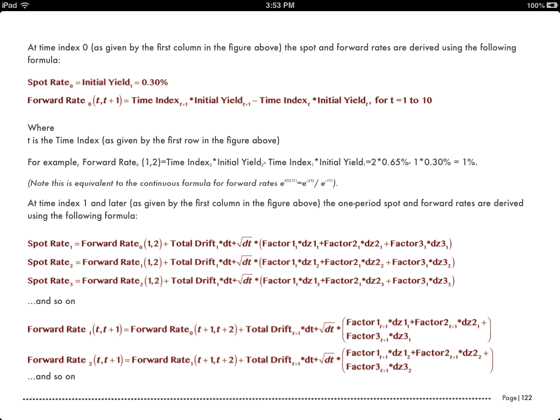•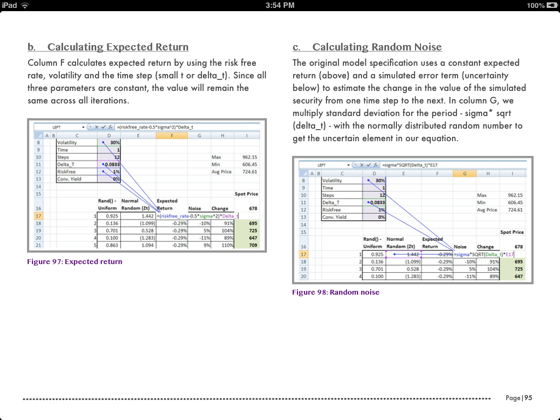•